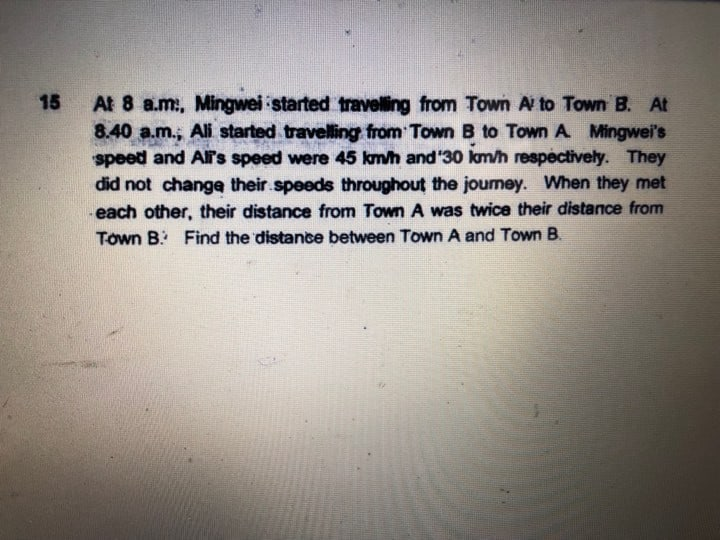# Questionhelp pls…

When A starts,  M has already traveled (40÷60) x 45 = 30 km

A—-X————o——–B

30 + 45 h = 2 x 30h

30 + 45 h = 60 h

30 = 60h -45h

15h = 30

h = 2

Therefore, the distance is

30 + (45+30)x2 = 30 + 75×2 = 30 + 150 =180

0 Replies 0 Likes

Speed of M = 45 km/hr;  Speed of A = 30 km/hr

Ratio of speed M to speed A will be 3 : 2, therefore at a same time period, Ratio of distance travelled by M to distance travelled by A will also be 3 : 2

45 km/hr x 40/60 hr = 30 km

At 8.40am, M travelled 30 km from Town A

When they meet, A has travelled 2 units of distance from Town B, and M has travelled 30km + 3 units from Town A.

2 x 2 units = 30 km + 3 units

4 units = 30 km + 3 units

1 unit = 30 km.

Total distance from Town A to Town B will be,

5 units + 30 km = (5 x 30)km + 30 km = 180 km

0 Replies 0 Likes